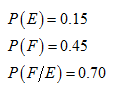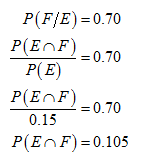# suppose that p(E) = .15, p(F) = .45, and p(F/E) = .70. question: What is p(E/F)?

Question
1 views

suppose that p(E) = .15, p(F) = .45, and p(F/E) = .70.

question: What is p(E/F)?

check_circle

Step 1

Solution:

From the given information,Then,...

### Want to see the full answer?

See Solution

#### Want to see this answer and more?

Solutions are written by subject experts who are available 24/7. Questions are typically answered within 1 hour.*

See Solution
*Response times may vary by subject and question.
Tagged in